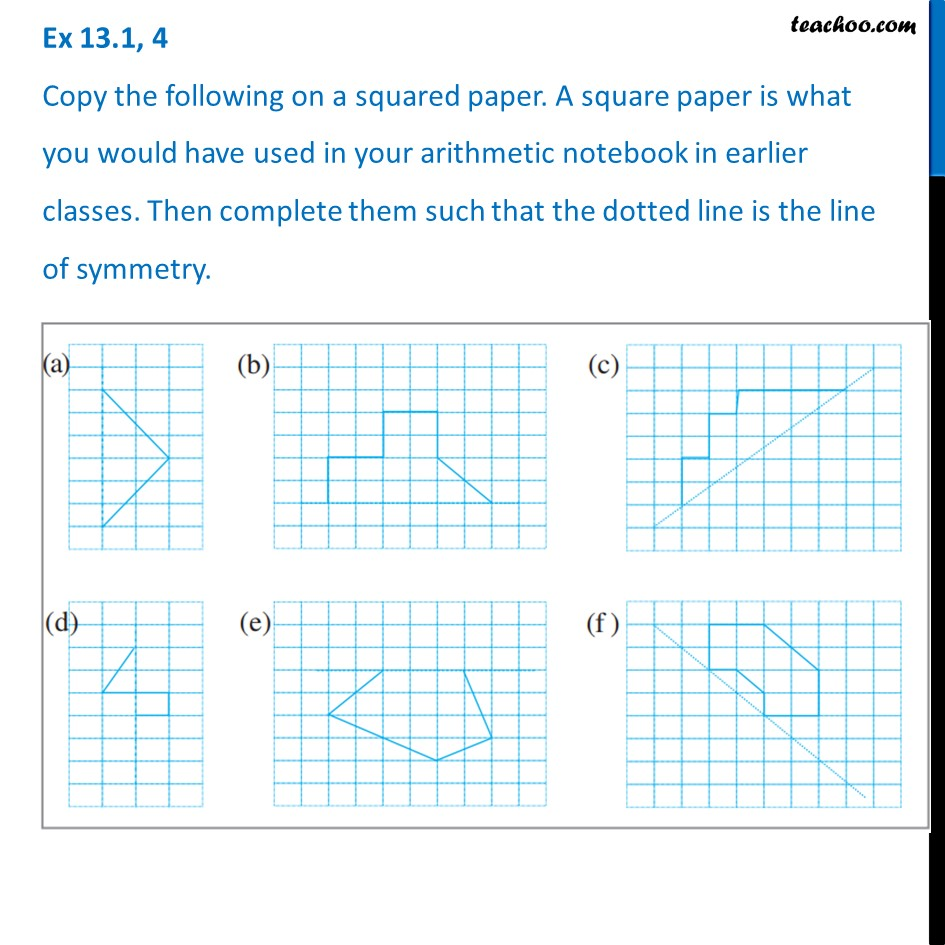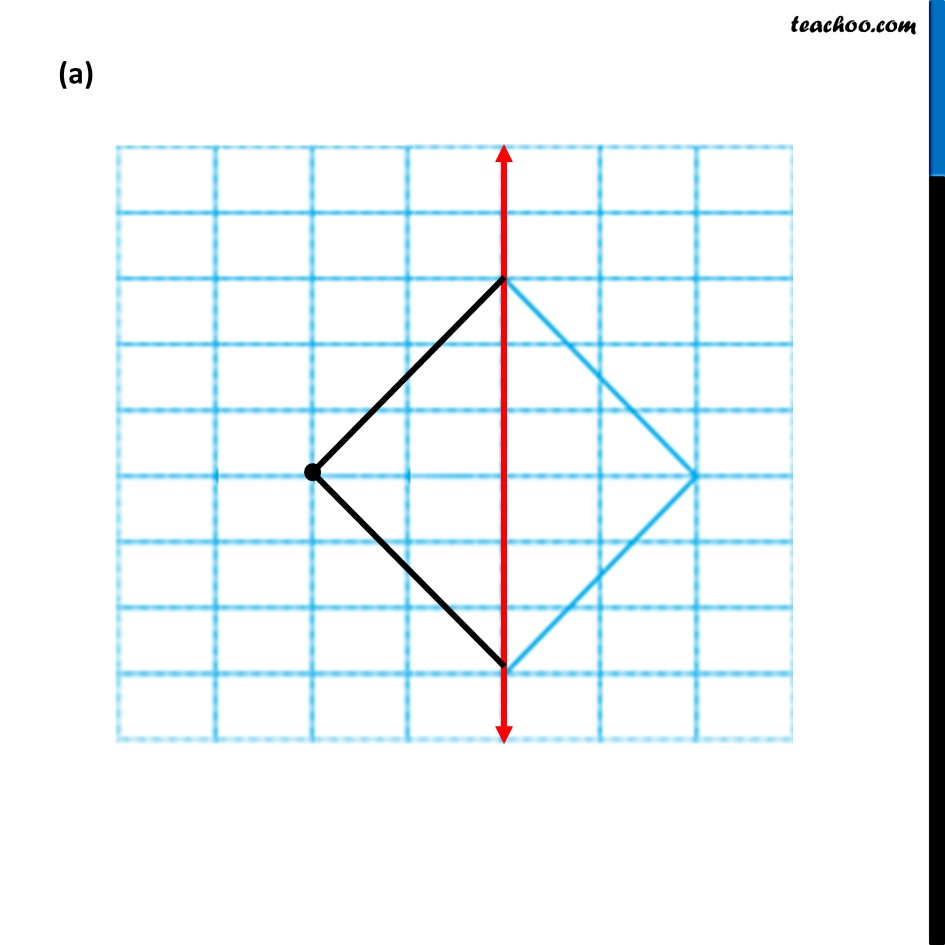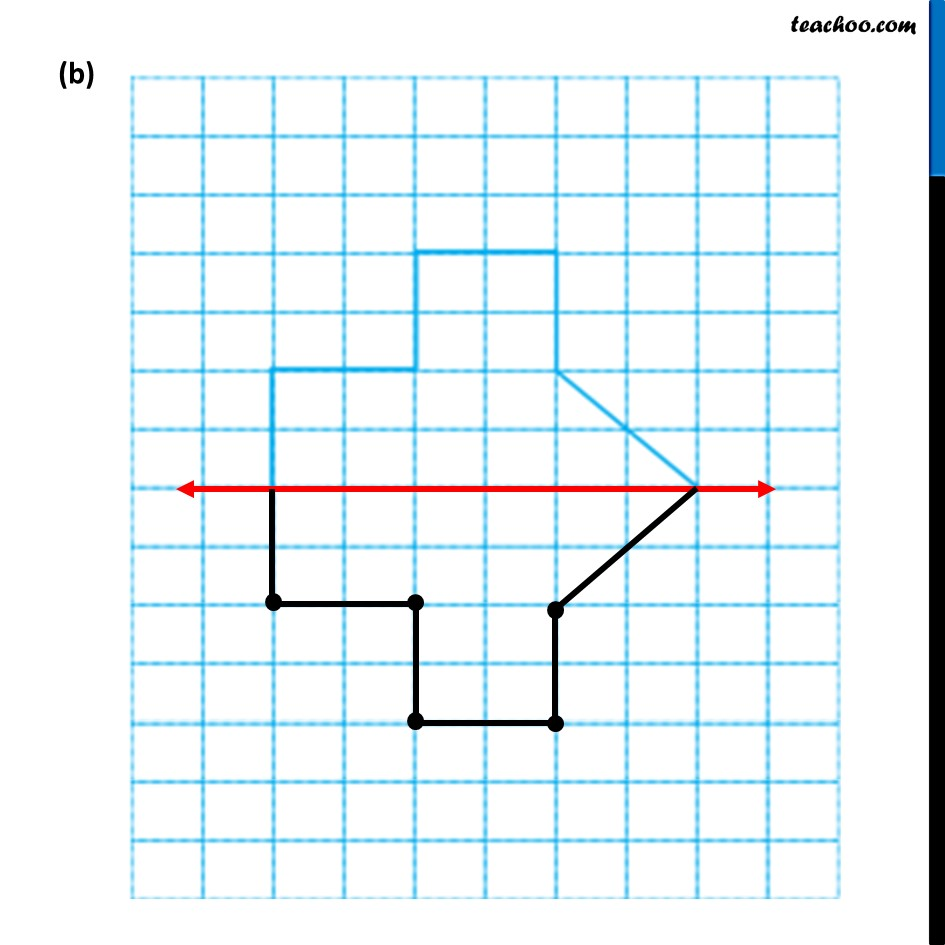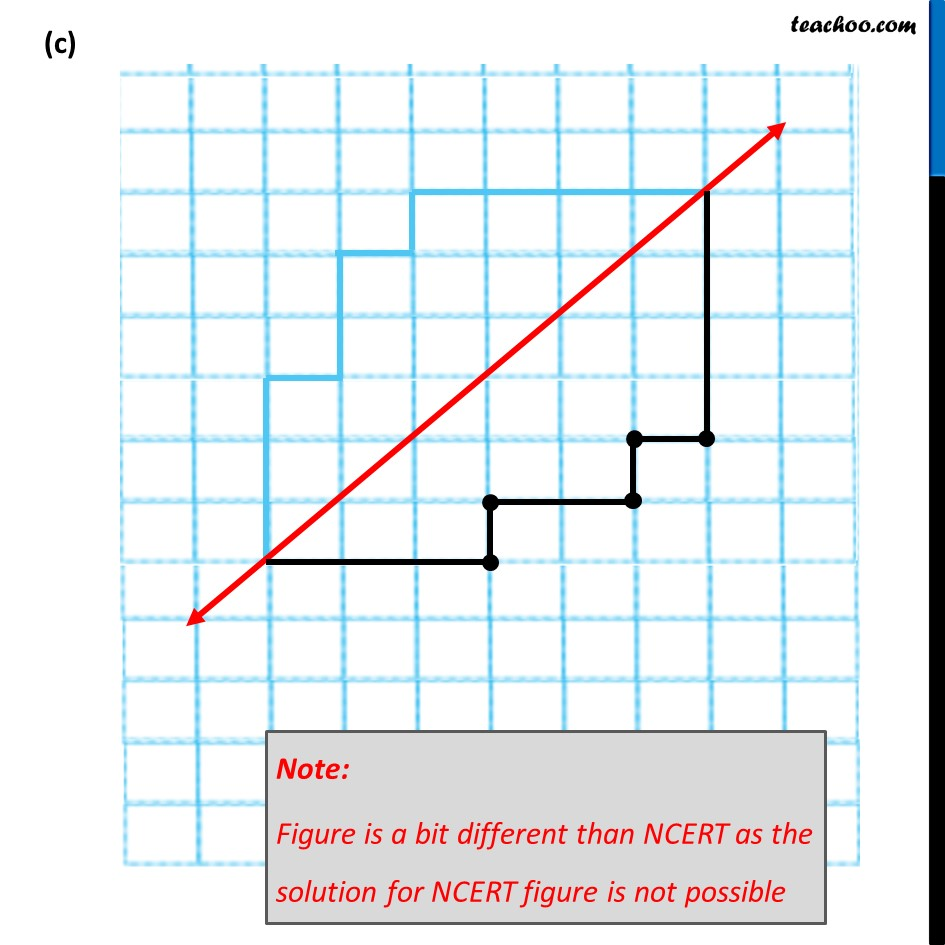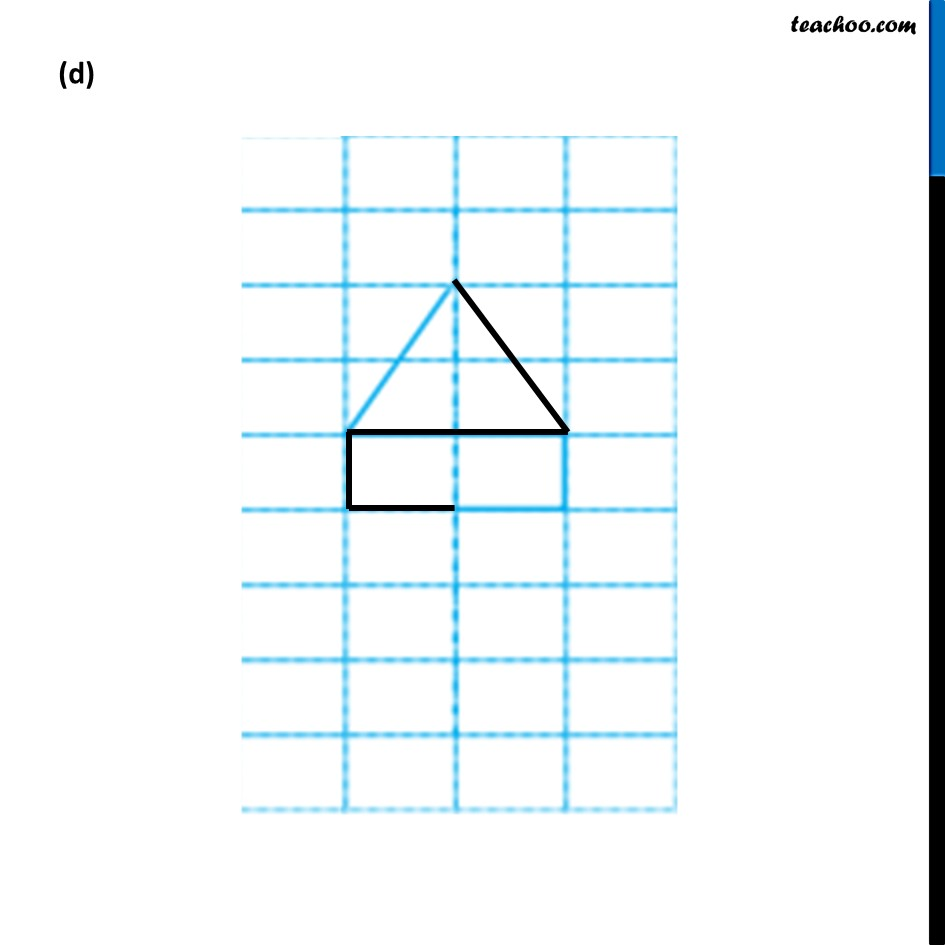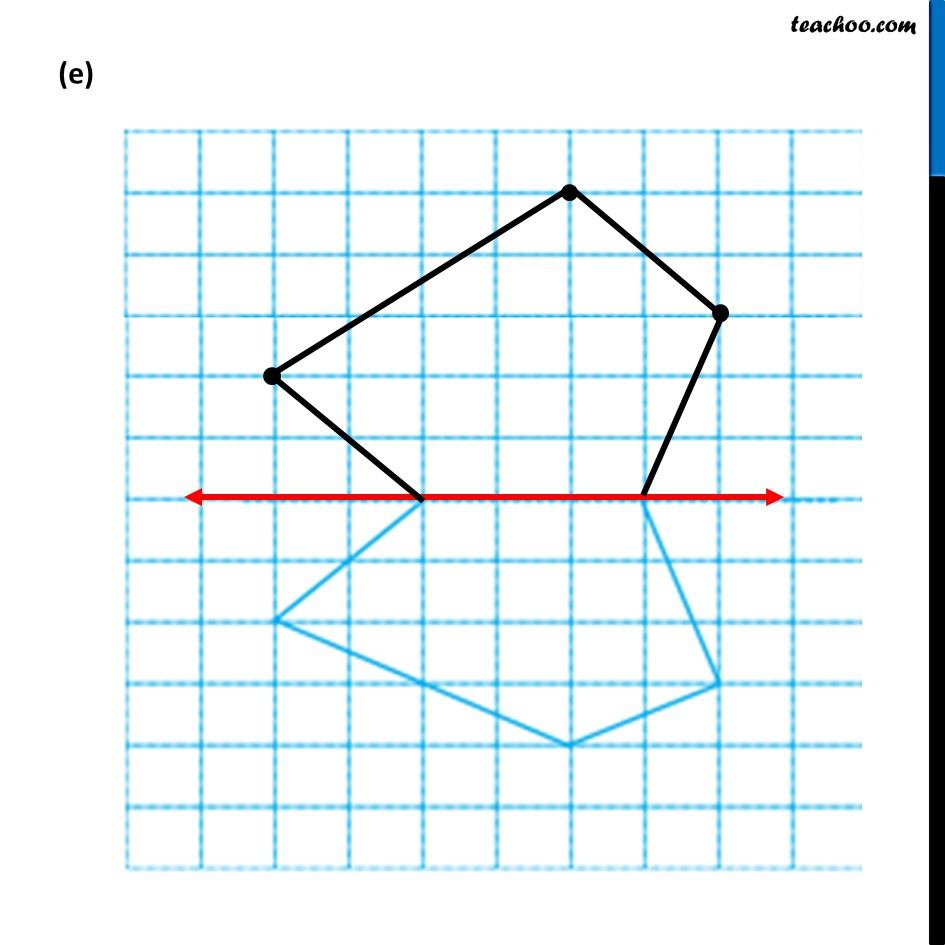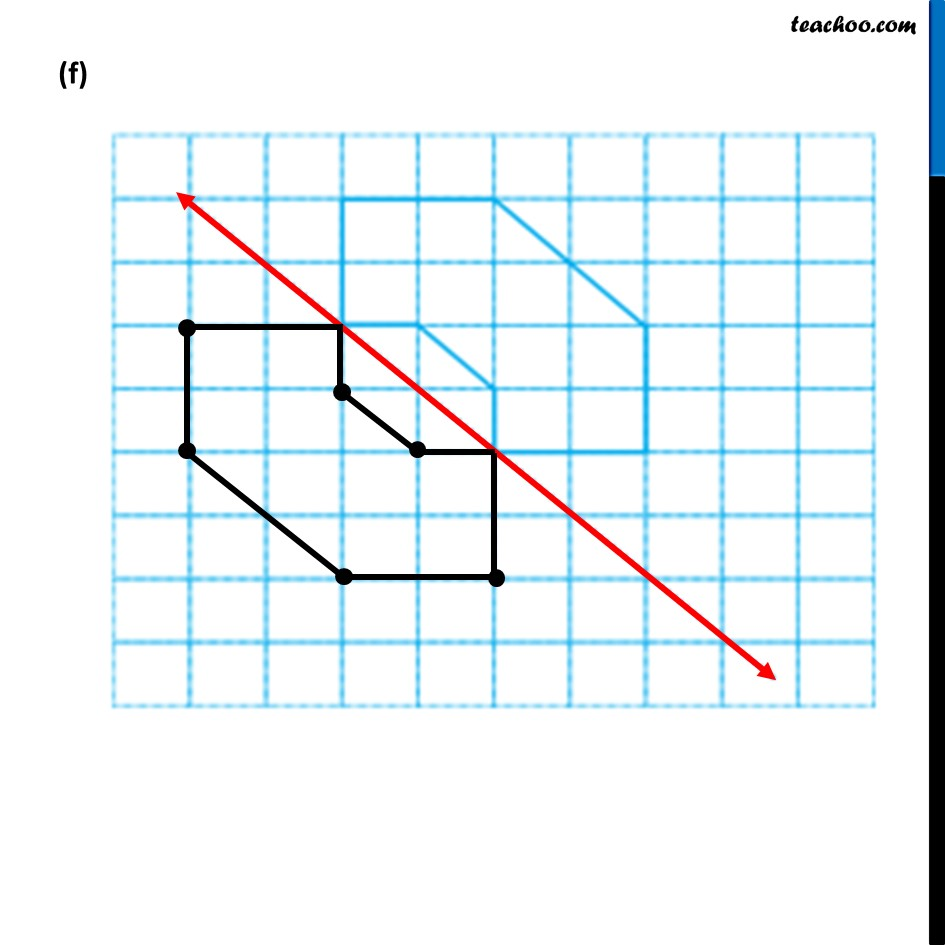1. Chapter 13 Class 6 Symmetry
2. Serial order wise
3. Ex 13.1

Transcript

Ex 13.1, 4 Copy the following on a squared paper. A square paper is what you would have used in your arithmetic notebook in earlier classes. Then complete them such that the dotted line is the line of symmetry.

Ex 13.1

Chapter 13 Class 6 Symmetry
Serial order wise